Calculus Volume 3

# 2.2Vectors in Three Dimensions

Calculus Volume 32.2 Vectors in Three Dimensions

### Learning Objectives

• 2.2.1 Describe three-dimensional space mathematically.
• 2.2.2 Locate points in space using coordinates.
• 2.2.3 Write the distance formula in three dimensions.
• 2.2.4 Write the equations for simple planes and spheres.
• 2.2.5 Perform vector operations in $ℝ 3 . ℝ 3 .$

Vectors are useful tools for solving two-dimensional problems. Life, however, happens in three dimensions. To expand the use of vectors to more realistic applications, it is necessary to create a framework for describing three-dimensional space. For example, although a two-dimensional map is a useful tool for navigating from one place to another, in some cases the topography of the land is important. Does your planned route go through the mountains? Do you have to cross a river? To appreciate fully the impact of these geographic features, you must use three dimensions. This section presents a natural extension of the two-dimensional Cartesian coordinate plane into three dimensions.

### Three-Dimensional Coordinate Systems

As we have learned, the two-dimensional rectangular coordinate system contains two perpendicular axes: the horizontal x-axis and the vertical y-axis. We can add a third dimension, the z-axis, which is perpendicular to both the x-axis and the y-axis. We call this system the three-dimensional rectangular coordinate system. It represents the three dimensions we encounter in real life.

### Definition

The three-dimensional rectangular coordinate system consists of three perpendicular axes: the x-axis, the y-axis, the z-axis, and an origin at the point of intersection (0) of the axes. Because each axis is a number line representing all real numbers in $ℝ,ℝ,$ the three-dimensional system is often denoted by $ℝ3.ℝ3.$

In Figure 2.23(a), the positive z-axis is shown above the plane containing the x- and y-axes. The positive x-axis appears to the left and the positive y-axis is to the right. A natural question to ask is: How was arrangement determined? The system displayed follows the right-hand rule. If we take our right hand and align the fingers with the positive x-axis, then curl the fingers so they point in the direction of the positive y-axis, our thumb points in the direction of the positive z-axis. In this text, we always work with coordinate systems set up in accordance with the right-hand rule. Some systems do follow a left-hand rule, but the right-hand rule is considered the standard representation.

Figure 2.23 (a) We can extend the two-dimensional rectangular coordinate system by adding a third axis, the z-axis, that is perpendicular to both the x-axis and the y-axis. (b) The right-hand rule is used to determine the placement of the coordinate axes in the standard Cartesian plane.

In two dimensions, we describe a point in the plane with the coordinates $(x,y).(x,y).$ Each coordinate describes how the point aligns with the corresponding axis. In three dimensions, a new coordinate, $z,z,$ is appended to indicate alignment with the z-axis: $(x,y,z).(x,y,z).$ A point in space is identified by all three coordinates (Figure 2.24). To plot the point $(x,y,z),(x,y,z),$ go x units along the x-axis, then $yy$ units in the direction of the y-axis, then $zz$ units in the direction of the z-axis.

Figure 2.24 To plot the point $(x,y,z)(x,y,z)$ go $xx$ units along the x-axis, then $yy$ units in the direction of the y-axis, then $zz$ units in the direction of the z-axis.

### Example 2.11

#### Locating Points in Space

Sketch the point $(1,−2,3)(1,−2,3)$ in three-dimensional space.

### Checkpoint2.11

Sketch the point $(−2,3,−1)(−2,3,−1)$ in three-dimensional space.

In two-dimensional space, the coordinate plane is defined by a pair of perpendicular axes. These axes allow us to name any location within the plane. In three dimensions, we define coordinate planes by the coordinate axes, just as in two dimensions. There are three axes now, so there are three intersecting pairs of axes. Each pair of axes forms a coordinate plane: the xy-plane, the xz-plane, and the yz-plane (Figure 2.26). We define the xy-plane formally as the following set: ${(x,y,0):x,y∈ℝ}.{(x,y,0):x,y∈ℝ}.$ Similarly, the xz-plane and the yz-plane are defined as ${(x,0,z):x,z∈ℝ}{(x,0,z):x,z∈ℝ}$ and ${(0,y,z):y,z∈ℝ},{(0,y,z):y,z∈ℝ},$ respectively.

To visualize this, imagine you’re building a house and are standing in a room with only two of the four walls finished. (Assume the two finished walls are adjacent to each other.) If you stand with your back to the corner where the two finished walls meet, facing out into the room, the floor is the xy-plane, the wall to your right is the xz-plane, and the wall to your left is the yz-plane.

Figure 2.26 The plane containing the x- and y-axes is called the xy-plane. The plane containing the x- and z-axes is called the xz-plane, and the y- and z-axes define the yz-plane.

In two dimensions, the coordinate axes partition the plane into four quadrants. Similarly, the coordinate planes divide space between them into eight regions about the origin, called octants. The octants fill $ℝ3ℝ3$ in the same way that quadrants fill $ℝ2,ℝ2,$ as shown in Figure 2.27.

Figure 2.27 Points that lie in octants have three nonzero coordinates.

Most work in three-dimensional space is a comfortable extension of the corresponding concepts in two dimensions. In this section, we use our knowledge of circles to describe spheres, then we expand our understanding of vectors to three dimensions. To accomplish these goals, we begin by adapting the distance formula to three-dimensional space.

If two points lie in the same coordinate plane, then it is straightforward to calculate the distance between them. We that the distance $dd$ between two points $(x1,y1)(x1,y1)$ and $(x2,y2)(x2,y2)$ in the xy-coordinate plane is given by the formula

$d=(x2−x1)2+(y2−y1)2.d=(x2−x1)2+(y2−y1)2.$

The formula for the distance between two points in space is a natural extension of this formula.

### Theorem2.2

#### The Distance between Two Points in Space

The distance $dd$ between points $(x1,y1,z1)(x1,y1,z1)$ and $(x2,y2,z2)(x2,y2,z2)$ is given by the formula

$d=(x2−x1)2+(y2−y1)2+(z2−z1)2.d=(x2−x1)2+(y2−y1)2+(z2−z1)2.$
(2.1)

The proof of this theorem is left as an exercise. (Hint: First find the distance $d1d1$ between the points $(x1,y1,z1)(x1,y1,z1)$ and $(x2,y2,z1)(x2,y2,z1)$ as shown in Figure 2.28.)

Figure 2.28 The distance between $P1P1$ and $P2P2$ is the length of the diagonal of the rectangular prism having $P1P1$ and $P2P2$ as opposite corners.

### Example 2.12

#### Distance in Space

Find the distance between points $P1=(3,−1,5)P1=(3,−1,5)$ and $P2=(2,1,−1).P2=(2,1,−1).$

Figure 2.29 Find the distance between the two points.

### Checkpoint2.12

Find the distance between points $P1=(1,−5,4)P1=(1,−5,4)$ and $P2=(4,−1,−1).P2=(4,−1,−1).$

Before moving on to the next section, let’s get a feel for how $ℝ3ℝ3$ differs from $ℝ2.ℝ2.$ For example, in $ℝ2,ℝ2,$ lines that are not parallel must always intersect. This is not the case in $ℝ3.ℝ3.$ For example, consider the line shown in Figure 2.30. These two lines are not parallel, nor do they intersect.

Figure 2.30 These two lines are not parallel, but still do not intersect.

You can also have circles that are interconnected but have no points in common, as in Figure 2.31.

Figure 2.31 These circles are interconnected, but have no points in common.

We have a lot more flexibility working in three dimensions than we do if we stick with only two dimensions.

### Writing Equations in ℝ3

Now that we can represent points in space and find the distance between them, we can learn how to write equations of geometric objects such as lines, planes, and curved surfaces in $ℝ3.ℝ3.$ First, we start with a simple equation. Compare the graphs of the equation $x=0x=0$ in $ℝ,ℝ2,andℝ3ℝ,ℝ2,andℝ3$ (Figure 2.32). From these graphs, we can see the same equation can describe a point, a line, or a plane.

Figure 2.32 (a) In $ℝ,ℝ,$ the equation $x=0x=0$ describes a single point. (b) In $ℝ2,ℝ2,$ the equation $x=0x=0$ describes a line, the y-axis. (c) In $ℝ3,ℝ3,$ the equation $x=0x=0$ describes a plane, the yz-plane.

In space, the equation $x=0x=0$ describes all points $(0,y,z).(0,y,z).$ This equation defines the yz-plane. Similarly, the xy-plane contains all points of the form $(x,y,0).(x,y,0).$ The equation $z=0z=0$ defines the xy-plane and the equation $y=0y=0$ describes the xz-plane (Figure 2.33).

Figure 2.33 (a) In space, the equation $z=0z=0$ describes the xy-plane. (b) All points in the xz-plane satisfy the equation $y=0.y=0.$

Understanding the equations of the coordinate planes allows us to write an equation for any plane that is parallel to one of the coordinate planes. When a plane is parallel to the xy-plane, for example, the z-coordinate of each point in the plane has the same constant value. Only the x- and y-coordinates of points in that plane vary from point to point.

### Rule: Equations of Planes Parallel to Coordinate Planes

1. The plane in space that is parallel to the xy-plane and contains point $(a,b,c)(a,b,c)$ can be represented by the equation $z=c.z=c.$
2. The plane in space that is parallel to the xz-plane and contains point $(a,b,c)(a,b,c)$ can be represented by the equation $y=b.y=b.$
3. The plane in space that is parallel to the yz-plane and contains point $(a,b,c)(a,b,c)$ can be represented by the equation $x=a.x=a.$

### Example 2.13

#### Writing Equations of Planes Parallel to Coordinate Planes

1. Write an equation of the plane passing through point $(3,11,7)(3,11,7)$ that is parallel to the yz-plane.
2. Find an equation of the plane passing through points $(6,−2,9),(6,−2,9),$ $(0,−2,4),(0,−2,4),$ and $(1,−2,−3).(1,−2,−3).$

### Checkpoint2.13

Write an equation of the plane passing through point $(1,−6,−4)(1,−6,−4)$ that is parallel to the xy-plane.

As we have seen, in $ℝ2ℝ2$ the equation $x=5x=5$ describes the vertical line passing through point $(5,0).(5,0).$ This line is parallel to the y-axis. In a natural extension, the equation $x=5x=5$ in $ℝ3ℝ3$ describes the plane passing through point $(5,0,0),(5,0,0),$ which is parallel to the yz-plane. Another natural extension of a familiar equation is found in the equation of a sphere.

### Definition

A sphere is the set of all points in space equidistant from a fixed point, the center of the sphere (Figure 2.34), just as the set of all points in a plane that are equidistant from the center represents a circle. In a sphere, as in a circle, the distance from the center to a point on the sphere is called the radius.

Figure 2.34 Each point $(x,y,z)(x,y,z)$ on the surface of a sphere is $rr$ units away from the center $(a,b,c).(a,b,c).$

The equation of a circle is derived using the distance formula in two dimensions. In the same way, the equation of a sphere is based on the three-dimensional formula for distance.

### Rule: Equation of a Sphere

The sphere with center $(a,b,c)(a,b,c)$ and radius $rr$ can be represented by the equation

$(x−a)2+(y−b)2+(z−c)2=r2.(x−a)2+(y−b)2+(z−c)2=r2.$
(2.2)

This equation is known as the standard equation of a sphere.

### Example 2.14

#### Finding an Equation of a Sphere

Find the standard equation of the sphere with center $(10,7,4)(10,7,4)$ and point $(−1,3,−2),(−1,3,−2),$ as shown in Figure 2.35.

Figure 2.35 The sphere centered at $(10,7,4)(10,7,4)$ containing point $(−1,3,−2).(−1,3,−2).$

### Checkpoint2.14

Find the standard equation of the sphere with center $(−2,4,−5)(−2,4,−5)$ containing point $(4,4,−1).(4,4,−1).$

### Example 2.15

#### Finding the Equation of a Sphere

Let $P=(−5,2,3)P=(−5,2,3)$ and $Q=(3,4,−1),Q=(3,4,−1),$ and suppose line segment $PQPQ$ forms the diameter of a sphere (Figure 2.36). Find the equation of the sphere.

Figure 2.36 Line segment $PQ.PQ.$

### Checkpoint2.15

Find the equation of the sphere with diameter $PQ,PQ,$ where $P=(2,−1,−3)P=(2,−1,−3)$ and $Q=(−2,5,−1).Q=(−2,5,−1).$

### Example 2.16

#### Graphing Other Equations in Three Dimensions

Describe the set of points that satisfies $(x−4)(z−2)=0,(x−4)(z−2)=0,$ and graph the set.

### Checkpoint2.16

Describe the set of points that satisfies $(y+2)(z−3)=0,(y+2)(z−3)=0,$ and graph the set.

### Example 2.17

#### Graphing Other Equations in Three Dimensions

Describe the set of points in three-dimensional space that satisfies $(x−2)2+(y−1)2=4,(x−2)2+(y−1)2=4,$ and graph the set.

### Checkpoint2.17

Describe the set of points in three dimensional space that satisfies $x2+(z−2)2=16,x2+(z−2)2=16,$ and graph the surface.

### Working with Vectors in ℝ3

Just like two-dimensional vectors, three-dimensional vectors are quantities with both magnitude and direction, and they are represented by directed line segments (arrows). With a three-dimensional vector, we use a three-dimensional arrow.

Three-dimensional vectors can also be represented in component form. The notation $v=〈x,y,z〉v=〈x,y,z〉$ is a natural extension of the two-dimensional case, representing a vector with the initial point at the origin, $(0,0,0),(0,0,0),$ and terminal point $(x,y,z).(x,y,z).$ The zero vector is $0=〈0,0,0〉.0=〈0,0,0〉.$ So, for example, the three dimensional vector $v=〈2,4,1〉v=〈2,4,1〉$ is represented by a directed line segment from point $(0,0,0)(0,0,0)$ to point $(2,4,1)(2,4,1)$ (Figure 2.39).

Figure 2.39 Vector $v=〈2,4,1〉v=〈2,4,1〉$ is represented by a directed line segment from point $(0,0,0)(0,0,0)$ to point $(2,4,1).(2,4,1).$

Vector addition and scalar multiplication are defined analogously to the two-dimensional case. If $v=〈x1,y1,z1〉v=〈x1,y1,z1〉$ and $w=〈x2,y2,z2〉w=〈x2,y2,z2〉$ are vectors, and $kk$ is a scalar, then

$v+w=〈x1+x2,y1+y2,z1+z2〉andkv=〈kx1,ky1,kz1〉.v+w=〈x1+x2,y1+y2,z1+z2〉andkv=〈kx1,ky1,kz1〉.$

If $k=−1,k=−1,$ then $kv=(−1)vkv=(−1)v$ is written as $−v,−v,$ and vector subtraction is defined by $v−w=v+(−w)=v+(−1)w.v−w=v+(−w)=v+(−1)w.$

The standard unit vectors extend easily into three dimensions as well—$i=〈1,0,0〉,i=〈1,0,0〉,$ $j=〈0,1,0〉,j=〈0,1,0〉,$ and $k=〈0,0,1〉k=〈0,0,1〉$—and we use them in the same way we used the standard unit vectors in two dimensions. Thus, we can represent a vector in $ℝ3ℝ3$ in the following ways:

$v=〈x,y,z〉=xi+yj+zk.v=〈x,y,z〉=xi+yj+zk.$

### Example 2.18

#### Vector Representations

Let $PQ→PQ→$ be the vector with initial point $P=(3,12,6)P=(3,12,6)$ and terminal point $Q=(−4,−3,2)Q=(−4,−3,2)$ as shown in Figure 2.40. Express $PQ→PQ→$ in both component form and using standard unit vectors.

Figure 2.40 The vector with initial point $P=(3,12,6)P=(3,12,6)$ and terminal point $Q=(−4,−3,2).Q=(−4,−3,2).$

### Checkpoint2.18

Let $S=(3,8,2)S=(3,8,2)$ and $T=(2,−1,3).T=(2,−1,3).$ Express $ST→ST→$ in component form and in standard unit form.

As described earlier, vectors in three dimensions behave in the same way as vectors in a plane. The geometric interpretation of vector addition, for example, is the same in both two- and three-dimensional space (Figure 2.41).

Figure 2.41 To add vectors in three dimensions, we follow the same procedures we learned for two dimensions.

We have already seen how some of the algebraic properties of vectors, such as vector addition and scalar multiplication, can be extended to three dimensions. Other properties can be extended in similar fashion. They are summarized here for our reference.

### Rule: Properties of Vectors in Space

Let $v=〈x1,y1,z1〉v=〈x1,y1,z1〉$ and $w=〈x2,y2,z2〉w=〈x2,y2,z2〉$ be vectors, and let $kk$ be a scalar.

Scalar multiplication: $kv=〈kx1,ky1,kz1〉kv=〈kx1,ky1,kz1〉$

Vector addition: $v+w=〈x1,y1,z1〉+〈x2,y2,z2〉=〈x1+x2,y1+y2,z1+z2〉v+w=〈x1,y1,z1〉+〈x2,y2,z2〉=〈x1+x2,y1+y2,z1+z2〉$

Vector subtraction: $v−w=〈x1,y1,z1〉−〈x2,y2,z2〉=〈x1−x2,y1−y2,z1−z2〉v−w=〈x1,y1,z1〉−〈x2,y2,z2〉=〈x1−x2,y1−y2,z1−z2〉$

Vector magnitude: $‖v‖=x12+y12+z12‖v‖=x12+y12+z12$

Unit vector in the direction of v: $1‖v‖v=1‖v‖〈x1,y1,z1〉=〈x1‖v‖,y1‖v‖,z1‖v‖〉,1‖v‖v=1‖v‖〈x1,y1,z1〉=〈x1‖v‖,y1‖v‖,z1‖v‖〉,$ if $v≠0v≠0$

We have seen that vector addition in two dimensions satisfies the commutative, associative, and additive inverse properties. These properties of vector operations are valid for three-dimensional vectors as well. Scalar multiplication of vectors satisfies the distributive property, and the zero vector acts as an additive identity. The proofs to verify these properties in three dimensions are straightforward extensions of the proofs in two dimensions.

### Example 2.19

#### Vector Operations in Three Dimensions

Let $v=〈−2,9,5〉v=〈−2,9,5〉$ and $w=〈1,−1,0〉w=〈1,−1,0〉$ (Figure 2.42). Find the following vectors.

1. $3v−2w3v−2w$
2. $5‖w‖5‖w‖$
3. $‖5w‖‖5w‖$
4. A unit vector in the direction of $vv$
Figure 2.42 The vectors $v=〈−2,9,5〉v=〈−2,9,5〉$ and $w=〈1,−1,0〉.w=〈1,−1,0〉.$

### Checkpoint2.19

Let $v=〈−1,−1,1〉v=〈−1,−1,1〉$ and $w=〈2,0,1〉.w=〈2,0,1〉.$ Find a unit vector in the direction of $5v+3w.5v+3w.$

### Example 2.20

#### Throwing a Forward Pass

A quarterback is standing on the football field preparing to throw a pass. His receiver is standing 20 yd down the field and 15 yd to the quarterback’s left. The quarterback throws the ball at a velocity of 60 mph toward the receiver at an upward angle of $30°30°$ (see the following figure). Write the initial velocity vector of the ball, $v,v,$ in component form.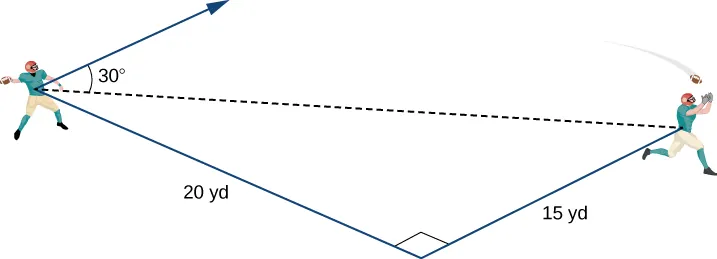### Checkpoint2.20

Assume the quarterback and the receiver are in the same place as in the previous example. This time, however, the quarterback throws the ball at velocity of $4040$ mph and an angle of $45°.45°.$ Write the initial velocity vector of the ball, $v,v,$ in component form.

### Section 2.2 Exercises

61.

Consider a rectangular box with one of the vertices at the origin, as shown in the following figure. If point $A(2,3,5)A(2,3,5)$ is the opposite vertex to the origin, then find

1. the coordinates of the other six vertices of the box and
2. the length of the diagonal of the box determined by the vertices $OO$ and $A.A.$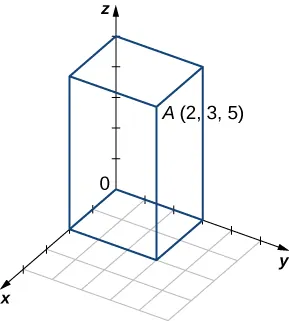62.

Find the coordinates of point $PP$ and determine its distance to the origin.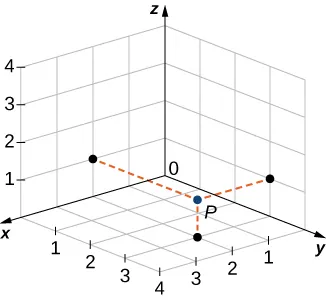For the following exercises, describe and graph the set of points that satisfies the given equation.

63.

$( y − 5 ) ( z − 6 ) = 0 ( y − 5 ) ( z − 6 ) = 0$

64.

$( z − 2 ) ( z − 5 ) = 0 ( z − 2 ) ( z − 5 ) = 0$

65.

$( y − 1 ) 2 + ( z − 1 ) 2 = 1 ( y − 1 ) 2 + ( z − 1 ) 2 = 1$

66.

$( x − 2 ) 2 + ( z − 5 ) 2 = 4 ( x − 2 ) 2 + ( z − 5 ) 2 = 4$

67.

Write the equation of the plane passing through point $(1,1,1)(1,1,1)$ that is parallel to the xy-plane.

68.

Write the equation of the plane passing through point $(1,−3,2)(1,−3,2)$ that is parallel to the xz-plane.

69.

Find an equation of the plane passing through points $(1,−3,−2),(1,−3,−2),$ $(0,3,−2),(0,3,−2),$ and $(1,0,−2).(1,0,−2).$

70.

Find an equation of the plane passing through points $(1,9,2),(1,9,2),$ $(1,3,6),(1,3,6),$ and $(1,−7,8).(1,−7,8).$

For the following exercises, find the equation of the sphere in standard form that satisfies the given conditions.

71.

Center $C(−1,7,4)C(−1,7,4)$ and radius $44$

72.

Center $C(−4,7,2)C(−4,7,2)$ and radius $66$

73.

Diameter $PQ,PQ,$ where $P(−1,5,7)P(−1,5,7)$ and $Q(−5,2,9)Q(−5,2,9)$

74.

Diameter $PQ,PQ,$ where $P(−16,−3,9)P(−16,−3,9)$ and $Q(−2,3,5)Q(−2,3,5)$

For the following exercises, find the center and radius of the sphere with an equation in general form that is given.

75.

$x 2 + y 2 + z 2 − 4 z + 3 = 0 x 2 + y 2 + z 2 − 4 z + 3 = 0$

76.

$x 2 + y 2 + z 2 − 6 x + 8 y − 10 z + 25 = 0 x 2 + y 2 + z 2 − 6 x + 8 y − 10 z + 25 = 0$

For the following exercises, express vector $PQ→PQ→$ with the initial point at $PP$ and the terminal point at $QQ$

1. in component form and
2. by using standard unit vectors.
77.

$P(3,0,2)P(3,0,2)$ and $Q(−1,−1,4)Q(−1,−1,4)$

78.

$P(0,10,5)P(0,10,5)$ and $Q(1,1,−3)Q(1,1,−3)$

79.

$P(−2,5,−8)P(−2,5,−8)$ and $M(1,−7,4),M(1,−7,4),$ where $MM$ is the midpoint of the line segment $PQPQ$

80.

$Q(0,7,−6)Q(0,7,−6)$ and $M(−1,3,2),M(−1,3,2),$ where $MM$ is the midpoint of the line segment $PQPQ$

81.

Find terminal point $QQ$ of vector $PQ→=〈7,−1,3〉PQ→=〈7,−1,3〉$ with the initial point at $P(−2,3,5).P(−2,3,5).$

82.

Find initial point $PP$ of vector $PQ→=〈−9,1,2〉PQ→=〈−9,1,2〉$ with the terminal point at $Q(10,0,−1).Q(10,0,−1).$

For the following exercises, use the given vectors $aa$ and $bb$ to find and express the vectors $a+b,a+b,$ $4a,4a,$ and $−5a+3b−5a+3b$ in component form.

83.

$a=〈−1,−2,4〉,a=〈−1,−2,4〉,$ $b=〈−5,6,−7〉b=〈−5,6,−7〉$

84.

$a=〈3,−2,4〉,a=〈3,−2,4〉,$ $b=〈−5,6,−9〉b=〈−5,6,−9〉$

85.

$a=−k,a=−k,$ $b=−ib=−i$

86.

$a=i+j+k,a=i+j+k,$ $b=2i−3j+2kb=2i−3j+2k$

For the following exercises, vectors u and v are given. Find the magnitudes of vectors $u−vu−v$ and $−2u.−2u.$

87.

$u=2i+3j+4k,u=2i+3j+4k,$ $v=−i+5j−kv=−i+5j−k$

88.

$u=i+j,u=i+j,$ $v=j−kv=j−k$

89.

$u=〈2cost,−2sint,3〉,u=〈2cost,−2sint,3〉,$ $v=〈0,0,3〉,v=〈0,0,3〉,$ where $tt$ is a real number.

90.

$u=〈0,1,sinht〉,u=〈0,1,sinht〉,$ $v=〈1,1,0〉,v=〈1,1,0〉,$ where $tt$ is a real number.

For the following exercises, find the unit vector in the direction of the given vector $aa$ and express it using standard unit vectors.

91.

$a = 3 i − 4 j a = 3 i − 4 j$

92.

$a = 〈 4 , −3 , 6 〉 a = 〈 4 , −3 , 6 〉$

93.

$a=PQ→,a=PQ→,$ where $P(−2,3,1)P(−2,3,1)$ and $Q(0,−4,4)Q(0,−4,4)$

94.

$a=OP→,a=OP→,$ where $P(−1,−1,1)P(−1,−1,1)$

95.

$a=u−v+w,a=u−v+w,$ where $u=i−j−k,u=i−j−k,$ $v=2i−j+k,v=2i−j+k,$ and $w=−i+j+3kw=−i+j+3k$

96.

$a=2u+v−w,a=2u+v−w,$ where $u=i−k,u=i−k,$ $v=2j,v=2j,$ and $w=i−jw=i−j$

97.

Determine whether $AB→AB→$ and $PQ→PQ→$ are equivalent vectors, where $A(1,1,1),B(3,3,3),P(1,4,5),A(1,1,1),B(3,3,3),P(1,4,5),$ and $Q(3,6,7).Q(3,6,7).$

98.

Determine whether the vectors $AB→AB→$ and $PQ→PQ→$ are equivalent, where $A(1,4,1),A(1,4,1),$ $B(−2,2,0),B(−2,2,0),$ $P(2,5,7),P(2,5,7),$ and $Q(−3,2,1).Q(−3,2,1).$

For the following exercises, find vector $uu$ with a magnitude that is given and satisfies the given conditions.

99.

$v=〈7,−1,3〉,v=〈7,−1,3〉,$ $‖u‖=10,‖u‖=10,$ $uu$ and $vv$ have the same direction

100.

$v=〈2,4,1〉,v=〈2,4,1〉,$ $‖u‖=15,‖u‖=15,$ $uu$ and $vv$ have the same direction

101.

$v=〈2sint,2cost,1〉,v=〈2sint,2cost,1〉,$ $‖u‖=2,‖u‖=2,$ $uu$ and $vv$ have opposite directions for any $t,t,$ where $tt$ is a real number

102.

$v=〈3sinht,0,3〉,v=〈3sinht,0,3〉,$ $‖u‖=5,‖u‖=5,$ $uu$ and $vv$ have opposite directions for any $t,t,$ where $tt$ is a real number

103.

Determine a vector of magnitude $55$ in the direction of vector $AB→,AB→,$ where $A(2,1,5)A(2,1,5)$ and $B(3,4,−7).B(3,4,−7).$

104.

Find a vector of magnitude $22$ that points in the opposite direction than vector $AB→,AB→,$ where $A(−1,−1,1)A(−1,−1,1)$ and $B(0,1,1).B(0,1,1).$ Express the answer in component form.

105.

Consider the points $A(2,α,0),B(0,1,β),A(2,α,0),B(0,1,β),$ and $C(1,1,β),C(1,1,β),$ where $αα$ and $ββ$ are negative real numbers. Find $αα$ and $ββ$ such that $‖OA→−OB→+OC→‖=‖OB→‖=4.‖OA→−OB→+OC→‖=‖OB→‖=4.$

106.

Consider points $A(α,0,0),B(0,β,0),A(α,0,0),B(0,β,0),$ and $C(α,β,β),C(α,β,β),$ where $αα$ and $ββ$ are positive real numbers. Find $αα$ and $ββ$ such that $‖OA—+OB—‖=2and‖OC—‖=3.‖OA—+OB—‖=2and‖OC—‖=3.$

107.

Let $P(x,y,z)P(x,y,z)$ be a point situated at an equal distance from points $A(1,−1,0)A(1,−1,0)$ and $B(−1,2,1).B(−1,2,1).$ Show that point $PP$ lies on the plane of equation $−2x+3y+z=2.−2x+3y+z=2.$

108.

Let $P(x,y,z)P(x,y,z)$ be a point situated at an equal distance from the origin and point $A(4,1,2).A(4,1,2).$ Show that the coordinates of point $PP$ satisfy the equation $8x+2y+4z=21.8x+2y+4z=21.$

109.

The points $A,B,A,B,$ and $CC$ are collinear (in this order) if the relation $‖AB→‖+‖BC→‖=‖AC→‖‖AB→‖+‖BC→‖=‖AC→‖$ is satisfied. Show that $A(5,3,−1),A(5,3,−1),$ $B(−5,−3,1),B(−5,−3,1),$ and $C(−15,−9,3)C(−15,−9,3)$ are collinear points.

110.

Show that points $A(1,0,1),A(1,0,1),$ $B(0,1,1),B(0,1,1),$ and $C(1,1,1)C(1,1,1)$ are not collinear.

111.

[T] A force $FF$ of $50N50N$ acts on a particle in the direction of the vector $OP→,OP→,$ where $P(3,4,0).P(3,4,0).$

1. Express the force as a vector in component form.
2. Find the angle between force $FF$ and the positive direction of the x-axis. Express the answer in degrees rounded to the nearest integer.
112.

[T] A force $FF$ of $40N40N$ acts on a box in the direction of the vector $OP→,OP→,$ where $P(1,0,2).P(1,0,2).$

1. Express the force as a vector by using standard unit vectors.
2. Find the angle between force $FF$ and the positive direction of the x-axis.
113.

If $FF$ is a force that moves an object from point $P1(x1,y1,z1)P1(x1,y1,z1)$ to another point $P2(x2,y2,z2),P2(x2,y2,z2),$ then the displacement vector is defined as $D=(x2−x1)i+(y2−y1)j+(z2−z1)k.D=(x2−x1)i+(y2−y1)j+(z2−z1)k.$ A metal container is lifted $1010$ m vertically by a constant force $F.F.$ Express the displacement vector $DD$ by using standard unit vectors.

114.

A box is pulled $44$ yd horizontally in the x-direction by a constant force $F.F.$ Find the displacement vector in component form.

115.

The sum of the forces acting on an object is called the resultant or net force. An object is said to be in static equilibrium if the resultant force of the forces that act on it is zero. Let $F1=〈10,6,3〉,F1=〈10,6,3〉,$ $F2=〈0,4,9〉,F2=〈0,4,9〉,$ and $F3=〈10,−3,−9〉F3=〈10,−3,−9〉$ be three forces acting on a box. Find the force $F4F4$ acting on the box such that the box is in static equilibrium. Express the answer in component form.

116.

[T] Let $Fk=〈1,k,k2〉,Fk=〈1,k,k2〉,$ $k=1,...,nk=1,...,n$ be $nn$ forces acting on a particle, with $n≥2.n≥2.$

1. Find the net force $F=∑k=1nFk.F=∑k=1nFk.$ Express the answer using standard unit vectors.
2. Use a computer algebra system (CAS) to find n such that $‖F‖<100.‖F‖<100.$
117.

The force of gravity $FF$ acting on an object is given by $F=mg,F=mg,$ where m is the mass of the object (expressed in kilograms) and $gg$ is acceleration resulting from gravity, with $‖g‖=9.8‖g‖=9.8$ $N/kg.N/kg.$ A 2-kg disco ball hangs by a chain from the ceiling of a room.

1. Find the force of gravity $FF$ acting on the disco ball and find its magnitude.
2. Find the force of tension $TT$ in the chain and its magnitude.
Express the answers using standard unit vectors.
Figure 2.43 (credit: modification of work by Kenneth Lu, Flickr)
118.

A 5-kg pendant chandelier is designed such that the alabaster bowl is held by four chains of equal length, as shown in the following figure.

1. Find the magnitude of the force of gravity acting on the chandelier.
2. Find the magnitudes of the forces of tension for each of the four chains (assume chains are essentially vertical).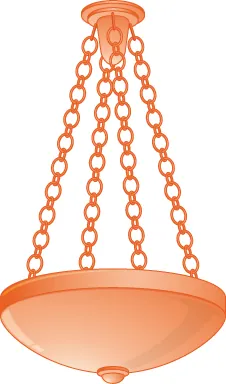119.

[T] A 30-kg block of cement is suspended by three cables of equal length that are anchored at points $P(−2,0,0),P(−2,0,0),$ $Q(1,3,0),Q(1,3,0),$ and $R(1,−3,0).R(1,−3,0).$ The load is located at $S(0,0,−23),S(0,0,−23),$ as shown in the following figure. Let $F1,F1,$ $F2,F2,$ and $F3F3$ be the forces of tension resulting from the load in cables $RS,QS,RS,QS,$ and $PS,PS,$ respectively.

1. Find the gravitational force $FF$ acting on the block of cement that counterbalances the sum $F1+F2+F3F1+F2+F3$ of the forces of tension in the cables.
2. Find forces $F1,F1,$ $F2,F2,$ and $F3.F3.$ Express the answer in component form.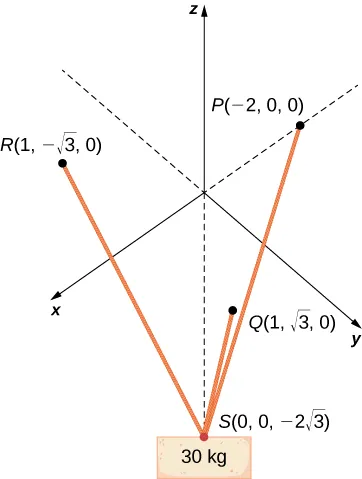120.

Two soccer players are practicing for an upcoming game. One of them runs 10 m from point A to point B. She then turns left at $90°90°$ and runs 10 m until she reaches point C. Then she kicks the ball with a speed of 10 m/sec at an upward angle of $45°45°$ to her teammate, who is located at point A. Write the velocity of the ball in component form.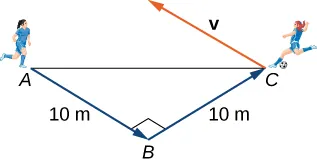121.

Let $r(t)=〈x(t),y(t),z(t)〉r(t)=〈x(t),y(t),z(t)〉$ be the position vector of a particle at the time $t∈[0,T],t∈[0,T],$ where $x,y,x,y,$ and $zz$ are smooth functions on $[0,T].[0,T].$ The instantaneous velocity of the particle at time $tt$ is defined by vector $v(t)=〈x′(t),y′(t),z′(t)〉,v(t)=〈x′(t),y′(t),z′(t)〉,$ with components that are the derivatives with respect to $t,t,$ of the functions x, y, and z, respectively. The magnitude $‖v(t)‖‖v(t)‖$ of the instantaneous velocity vector is called the speed of the particle at time t. Vector $a(t)=〈x″(t),y″(t),z″(t)〉,a(t)=〈x″(t),y″(t),z″(t)〉,$ with components that are the second derivatives with respect to $t,t,$ of the functions $x,y,x,y,$ and $z,z,$ respectively, gives the acceleration of the particle at time $t.t.$ Consider $r(t)=〈cost,sint,2t〉r(t)=〈cost,sint,2t〉$ the position vector of a particle at time $t∈[0,30],t∈[0,30],$ where the components of $rr$ are expressed in centimeters and time is expressed in seconds.

1. Find the instantaneous velocity, speed, and acceleration of the particle after the first second. Round your answer to two decimal places.
2. Use a CAS to visualize the path of the particle—that is, the set of all points of coordinates $(cost,sint,2t),(cost,sint,2t),$ where $t∈[0,30].t∈[0,30].$
122.

[T] Let $r(t)=〈t,2t2,4t2〉r(t)=〈t,2t2,4t2〉$ be the position vector of a particle at time $tt$ (in seconds), where $t∈[0,10]t∈[0,10]$ (here the components of $rr$ are expressed in centimeters).

1. Find the instantaneous velocity, speed, and acceleration of the particle after the first two seconds. Round your answer to two decimal places.
2. Use a CAS to visualize the path of the particle defined by the points $(t,2t2,4t2),(t,2t2,4t2),$ where $t∈[0,60].t∈[0,60].$
Order a print copy

As an Amazon Associate we earn from qualifying purchases.